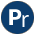#random.pl -- Random numbers

This library is derived from the DEC10 library random. Later, the core random generator was moved to C. The current version uses the SWI-Prolog arithmetic functions to realise this library. These functions are based on the GMP library.

author
- R.A. O'Keefe, V.S. Costa, L. Damas, Jan Wielemaker
- Built-in function random/1: A is `random(10)`
random(-R:float) is det
Binds R to a new random float in the open interval (0.0,1.0).
- setrand/1, getrand/1 may be used to fetch/set the state.
- In SWI-Prolog, random/1 is implemented by the function random_float/0.
random_between(+L:int, +U:int, -R:int) is semidet
Binds R to a random integer in [L,U] (i.e., including both L and U). Fails silently if U<L.
random(+L:int, +U:int, -R:int) is det
random(+L:float, +U:float, -R:float) is det
Generate a random integer or float in a range. If L and U are both integers, R is a random integer in the half open interval [L,U). If L and U are both floats, R is a float in the open interval (L,U).
deprecated
- Please use random/1 for generating a random float and random_between/3 for generating a random integer. Note that random_between/3 includes the upper bound, while this predicate excludes it.
setrand(+State) is det
getrand(-State) is det
Query/set the state of the random generator. This is intended for restarting the generator at a known state only. The predicate setrand/1 accepts an opaque term returned by getrand/1. This term may be asserted, written and read. The application may not make other assumptions about this term.

For compatibility reasons with older versions of this library, setrand/1 also accepts a term `rand(A,B,C)`, where A, B and C are integers in the range 1..30,000. This argument is used to seed the random generator. Deprecated.

Errors
- `existence_error(random_state, _)` is raised if the underlying infrastructure cannot fetch the random state. This is currently the case if SWI-Prolog is not compiled with the GMP library.
- set_random/1 and random_property/1 provide the SWI-Prolog native implementation.
maybe is semidet
Succeed/fail with equal probability (variant of maybe/1).
maybe(+P) is semidet
Succeed with probability P, fail with probability 1-P
maybe(+K, +N) is semidet
Succeed with probability K/N (variant of maybe/1)
random_perm2(?A, ?B, ?X, ?Y) is semidet
Does X=A,Y=B or X=B,Y=A with equal probability.
random_member(-X, +List:list) is semidet
X is a random member of List. Equivalent to random_between(1, |List|), followed by nth1/3. Fails of List is the empty list.
Compatibility
- Quintus and SICStus libraries.
random_select(-X, +List, -Rest) is semidet
random_select(+X, -List, +Rest) is det
Randomly select or insert an element. Either List or Rest must be a list. Fails if List is the empty list.
Compatibility
- Quintus and SICStus libraries.
random_subseq(+List, -Subseq, -Complement) is det
random_subseq(-List, +Subseq, +Complement) is semidet
Selects a random subsequence Subseq of List, with Complement containing all elements of List that were not selected. Each element of List is included with equal probability in either Subseq or Complement.

random_subseq/3 may also be called with Subseq and Complement bound and List unbound, which will recreate List by randomly interleaving Subseq and Complement. This mode may fail randomly, matching SICStus behavior. The failure probability corresponds to the probability of the "forward" mode selecting a Subseq/Complement combination with different lengths.

Compatibility
- SICStus 4
randset(+K:int, +N:int, -S:list(int)) is det
S is a sorted list of K unique random integers in the range 1..N. The implementation uses different techniques depending on the ratio K/N. For small K/N it generates a set of K random numbers, removes the duplicates and adds more numbers until |S| is K. For a large K/N it enumerates 1..N and decides randomly to include the number or not. For example:
```?- randset(5, 5, S).
S = [1, 2, 3, 4, 5].          (always)
?- randset(5, 20, S).
S = [2, 7, 10, 19, 20].```
- randseq/3.
randseq(+K:int, +N:int, -List:list(int)) is det
S is a list of K unique random integers in the range 1..N. The order is random. Defined as
```randseq(K, N, List) :-
randset(K, N, Set),
random_permutation(Set, List).```
- randset/3.
random_permutation(+List, -Permutation) is det
random_permutation(-List, +Permutation) is det
Permutation is a random permutation of List. This is intended to process the elements of List in random order. The predicate is symmetric.
Errors
- instantiation_error, `type_error(list, _)`.
random_numlist(+P, +L, +U, -List) is det
Unify List with an ascending list of integers between L and U (inclusive). Each integer in the range L..U is included with probability P.
Compatibility
- SICStus 4
partial_list(@Term) is semidet[private]
True if Term is a partial list.

## Undocumented predicates

The following predicates are exported, but not or incorrectly documented.

getrand(Arg1)